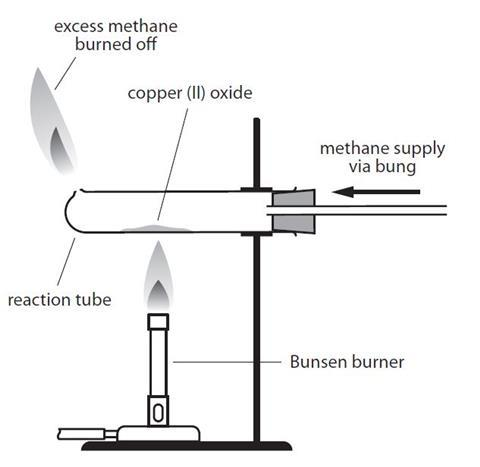Use this class practical with your students to deduce the formula of copper(II) oxide from its reduction by methane

In this experiment, students heat copper(II) oxide in a glass tube while passing methane over it, reducing the copper(II) oxide to copper. If they weigh the reactants and products carefully, students can then deduce the formula of the copper oxide. This can also be used simply as an example of reduction.

The practical is likely to take up to an hour, perhaps more to analyse the results. Students who have not carried out this type of reaction before may find it helpful to have the techniques demonstrated first. It is not really suitable for a class practical for students under the age of 14, but could be a useful demonstration.

Each pair or group of students will need access to two gas taps.

The students will need access to matches or lighters to light their Bunsen burners. Alternatively, light a few around the room and students can light their own using a splint.

## Equipment

### Apparatus

• Eye protection
• Reduction tube (hard glass test tube with small hole near closed end)
• 1-hole bung with glass tube to fit the reduction tube
• Rubber tubing
• Clamp stand, boss and clamp
• Bunsen burner
• Heat resistant mat
• Spatula
• Balance (must be accurate to at least 0.01 g)

### Chemicals

• Copper(II) oxide (HARMFUL, DANGEROUS FOR THE ENVIRONMENT), 2 spatulas
• Methane (natural gas) (EXTREMELY FLAMMABLE)

## Health, safety and technical notes

• Read our standard health and safety guidance.
• Wear eye protection throughout.
• Copper(II) oxide, CuO(s), (HARMFUL, DANGEROUS FOR THE ENVIRONMENT) – see CLEAPSS Hazcard HC026. For best results use analytical grade copper(II) oxide which has been dried by heating in an open dish at 300–400 °C for 10 min and then stored in a desiccator.
• Methane (natural gas), CH4 (g), (EXTREMELY FLAMMABLE) – see CLEAPSS Hazcard HC045a. It is likely that most school will be using a class gas tap for this experiment. This gas forms explosive mixtures in the air.
• It is also worth referring to the CLEAPSS Laboratory Handbook Section 13.2.3 for further information about this experiment.

## Procedure

1. Weigh the test tube with the bung in (mass 1). Put 2 spatulas of copper(II) oxide into the tube and spread it out as much as possible.
2. Weigh the tube again, with the copper oxide in it (mass 2).
3. Assemble the apparatus as shown in the diagram, but do not place the Bunsen burner underneath yet. Clamp the test tube as near to the bung as possible.Source: Royal Society of Chemistry

The equipment required for reducing copper(II) oxide using methane

1. Turn on the gas tap attached to the test tube about half way to get a steady flow of gas. This will pass methane through the apparatus.
2. Wait for at least 10 seconds, to allow all the air to be flushed out of the tube and then light the gas coming out of the hole at the end of the tube. If this experiment is a student activity, a teacher should supervise this step. Take care not to lean over the tube as you light the gas. Adjust the gas tap so that the flame is about 3 cm high.
3. Light the Bunsen burner and begin to heat the copper oxide in the tube. You will need to use a roaring flame (air hole fully open). You will need to pick up the Bunsen burner and move the flame around to heat every bit of the copper oxide. Ensure that the hottest part of the Bunsen burner flame (the top of the inner cone) is being used for heating. If there are parts which look unreacted, gently shake the tube – it will be very hot so do so by gently shaking the clamp stand.
4. When all the copper oxide looks like it has reacted (it will look like copper), keep heating for a further minute or two and then turn off the Bunsen burner.
5. Keep the methane passing over the product as it cools down to prevent it from reacting with any oxygen present and turning back into copper oxide. When the tube is cool, switch off the gas.
6. Weigh the test tube with the bung and the product (mass 3).

## Teaching notes

Students should have recorded the following masses:

• (mass 1) Test tube + bung
• (mass 2) Test tube + bung + copper oxide
• (mass 3) Test tube + bung + copper (product)

This should allow them to calculate the mass of the mass of the copper oxide (mass 2) – (mass 1) and the mass of the copper (mass 3) – (mass 1). They should also calculate the decrease in mass (mass 3) – (mass 2), which corresponds to the mass of oxygen.

With this information they can calculate the formula of the copper oxide.

Students will also need the relative atomic masses. Copper is 63.5 and oxygen is 16.

They should divide mass by the atomic mass for each element. This will give the number of moles of each.

Having done this for both elements, they should find the ratio between the two by dividing them both by the smallest number.

The ratio should be close to 1:1 as the formula of copper oxide is CuO.

### Example calculation

Mass copper oxide = 1.76 g

Mass copper = 1.43 g

So, mass oxygen = 0.33 g

Number moles Cu = 1.43/63.5 = 0.02251

Number moles O = 0.33/16 = 0.020625

Divide by the smallest to give the ratio approx. 1 Cu: 1 O

This would suggest a formula of CuO, which is the correct formula.